1 2 3

## Thermal analysis of a box-type solar cooker (1/2)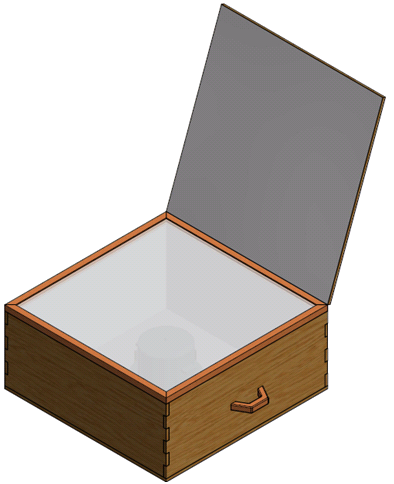I created a 3D model in order to estimate physical parameters of the solar cooker

The simplicity of solar cookers offers a lucrative alternative energy source to people who cannot afford expensive solar PV or solar water heaters, but are located in high irradiance areas. Areas in Africa are best candidates to make use of solar cookers, but the adoption of solar cookers has been hindered by misconception, small capacity and slowness . In this paper, a MATLAB model was developed, based on existing models, to investigate how advanced commercial products can be used to improve the performance of the solar cookers. Reducing the size of cooking fluid, applying commercial solar coatings to cooking vessel and optimal reflector tilt were found to significantly improve the performance of the solar cooker.

## Introduction

Compared to other solar collectors, solar cookers are simple to manufacture and inexpensive methods to harness solar thermal energy. Given the widespread energy shortages and high energy costs in most African states, one would expect that the adoption of solar cookers is widespread in Africa. However, this has not been true because of reasons that include slowness to cook traditional food, misconception, poor performance in winter, and lack of capacity to serve large families .

The most widely used solar cooker is the solar box-type cooker. The most general design of the box-type cooker consists of an enclosed metal or wooden box with a double-glass cover. Inside box, there are internal reflectors that reflect solar energy towards an absorber plate and or cooking vessel. The box-type cooker can come with an outside reflector to increase the solar energy reaching the cooking vessel as shown in Figure 1. Changing the designs and brands of components can significantly increase the acceptance and performance of solar box-type cookers. This paper seeks to use a simple mathematical model to identify commercial products that can be used to improve the performance of solar box-type cookers.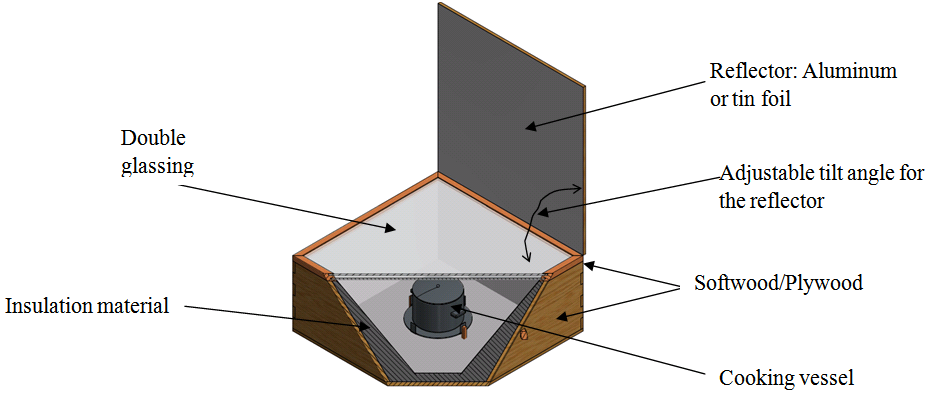Figure 1 A 3D model was created to estimate physical parameters of the solar cooker

## Methodology

After extensive literature review on solar box-type cookers and heat transfer processes, a simple solar cooker model consisting of 5 differential equations was setup in MATLAB. In addition to the mathematical model, a 3-D model was created using AutoCAD Inventor to estimate volume, mass, length and distances of solar cooker components and to generate illustrations.

A total of 8 scenarios were evaluated using MATLAB. In each scenario, selected parameters were changed while the rest of the parameters remained unchanged and similar to those in . Parameters were changed to match manufacturer datasheets of specific components of the solar cooker (e.g. glass transmittance). Variation of cooking fluid temperature with time was used as the basis for comparison.

## Mathematical Modeling

When heat is transferred to a body, enthalpy, H, of that body increases at a rate that is proportional to the rate of heat transfer. Enthalpy is the summation of the heat transfer and work done on a system. Change in enthalpy is proportional to temperature change for incompressible liquids, solids and ideal gases (equation 1) .

Where the middle term is the latent energy of the material associated with phase change. For this paper, it is assumed that all materials do not change phases so changes in enthalpy are proportional to changes in temperature as shown in equation 2 .

### Assumptions

#### Site Characterization

It was assumed that the solar cooker is used in Lawrence, MA, latitude,ϕ , of 42.717 ̊. Irradiance data was obtained from NREL’s TMY3 data as measured at Lawrence Municipality station . June 21 values of beam horizontal irradiance,J_bh, global horizontal irradiance, I_gh, and diffuse horizontal irradiance, I_d, and dry bulb temperature, T_a , were used to test the performance the solar cooker. Ambient temperature ranges from 14 ̊ C to 24 ̊ C whilst the irradiance varies as shown in Figure 2.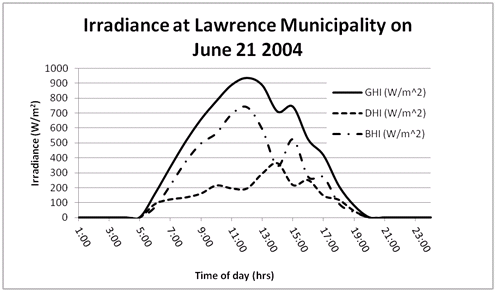#### Convective Heat Coefficients

For all convection heat transfer processes, natural convection was assumed in this paper. As long as the expression (Gr_L)/(Re_L^2 )≫1 is true, free convection can be assumed and so Nusselt number depends on Grashof and Prandtl numbers  . The exact relationship of Nusselt, Grashof and Prandtl was chosen based on whether there was turbulent or laminar flow . For laminar flow, average convective heat coefficient is given by the equation 2:

For where (10)^4<Gr*Pr<(10)^9 is true. When the flow becomes turbulent, then equation 4 can be used to obtain h_ave :

For where 10^9<Gr*Pr<10^13. Thermal properties of water and air were obtained from www.engineeringtoolbox.com.

In cases were one surface radiates heat to another, radiative view factors were assumed to be either two parallel disks or square plates as outlined in table 13.2 of . The view factor from the cooking vessel lid to the top surface of the cooking fluid was calculated based on two parallel circular disks. The view factor from lower glass to top glass was calculated based on two parallel square faces. Missing from the list view factor formulas is a view factor from a circular face to a square face; for this paper, such a view factor was assumed to be two parallel circular disks.

### Mathematical Model

The mathematical model was based on the paper by El-Sebaii with some minor modifications. Equations 5 to 11 were adapted from the same paper by El-Sebaii. . Instead of taking an analytical approach as in, this paper utilizes the power of MATLAB to find a time-dependant solutions.

The temperatures of the solar cooker were plotted in MATLAB using values from El-Sebaii as shown in shown Figure 4. In the figure, it is shown that the cooking fluid temperature continues to increase after reaching 100 ̊C, but in reality cooking fluid temperature flattens at 100 ̊C because any heat transmitted to the fluid causes change in latent heat of vaporization. However, an operator of a solar cooker can use a pressure cooker or cooking oil such that temperature of cooking fluid can reach temperatures higher than 100̊C.

In Figure 4 it is also shown that the cooking fluid temperature peaks about 6 hours after solar noon. This is likely because of the heat capacity and volume assigned to the cooking vessel and the cooking fluid.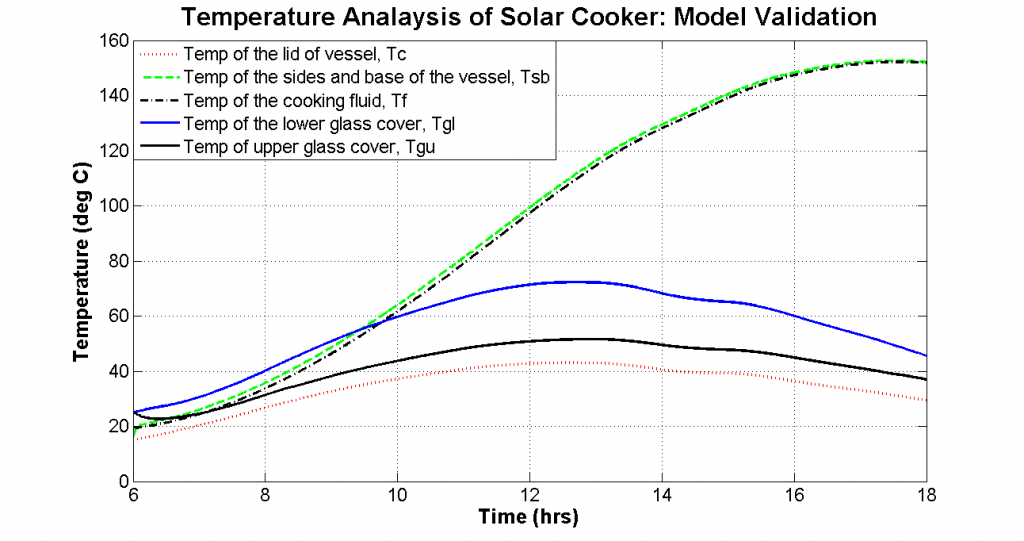Figure 4 The temperature plot of solar cooker to validate the mathematical model. In a practical situation, once the cooking fluid reaches 100 ̊ C, cooking fluid temperature remains constant because of latent heat of vaporization.

#### The vessel Lid

Rate of enthalpy change of the vessel lid is equivalent to the summation of the energy reflected into the box, energy directly incident on box’s glazing, heat loss to ambient air and heat loss to the cooking fluid .

Where F_cf and ε_c are new variables introduced in this paper to account for the radiation view factor from the lid to the cooking fluid and the emissivity of the lid. Numerical value of F_cf was determined from equations in a heat transfer book . Reflected radiation by the mirror/reflector is dependent on the view factor, Frg, of the reflector to the glazing and the reflectivity, ρ0, of the reflector .

#### Sides of the Cooking Vessel

Rate of enthalpy change of the sides of the vessel is equal to the summation of radiation incident on vessel walls, heat loss through convection to the fluid, heat loss through the base, and heat loss via sides of vessel .

The solar radiation that is reflected by the inner reflectors, Iri, depends on the reflectivity of the inner reflectors, overall optical efficiency of inner reflectors and the total irradiation through the glazing material .

#### The cooking fluid

Rate of enthalpy change of the cooking fluid is equal to the summation of radiative heat transfer from lid, convective heat transfer from vessel walls, heat loss via cooking vessel walls, convective transmission of the air gap, and heat loss through the top .

Rt is the combined thermal resistance of the fluid surface, top loss and conduction through cooking vessel .

#### Lower glass cover

Rate of enthalpy change for the lower glass cover is equal to the summation of the absorbed radiation, heat gain from the lead, heat gain from the cooking vessel walls, radiative heat loss to the upper glass, convective heat loss to top glass .

Where q_rcl and q_rlu are new terms introduced to account for heat radiated from the cover to the lower glass and heat radiated from the lower glass to the upper glass calculated as follows: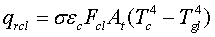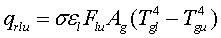(8, 9)

#### Upper glass cover

Rate of enthalpy change for the upper glass cover is equal to the summation of absorbed radiation, radiative heat gain from lower glass, convective heat gain from lower glass, radiative heat loss to ambient, and convective heat loss to the environment .

Where q_rua is a new term that is introduced to account for the radiative heat loss from the upper glass cover to the environment and is calculated as follows:

## Results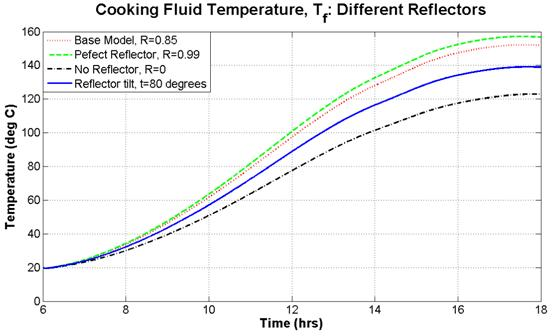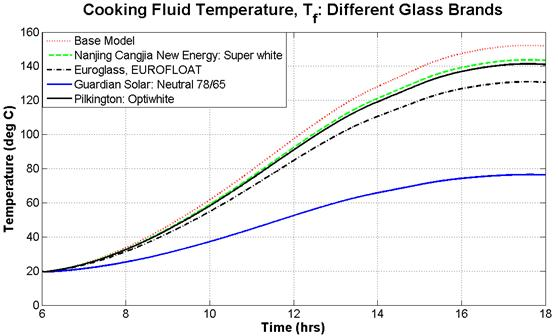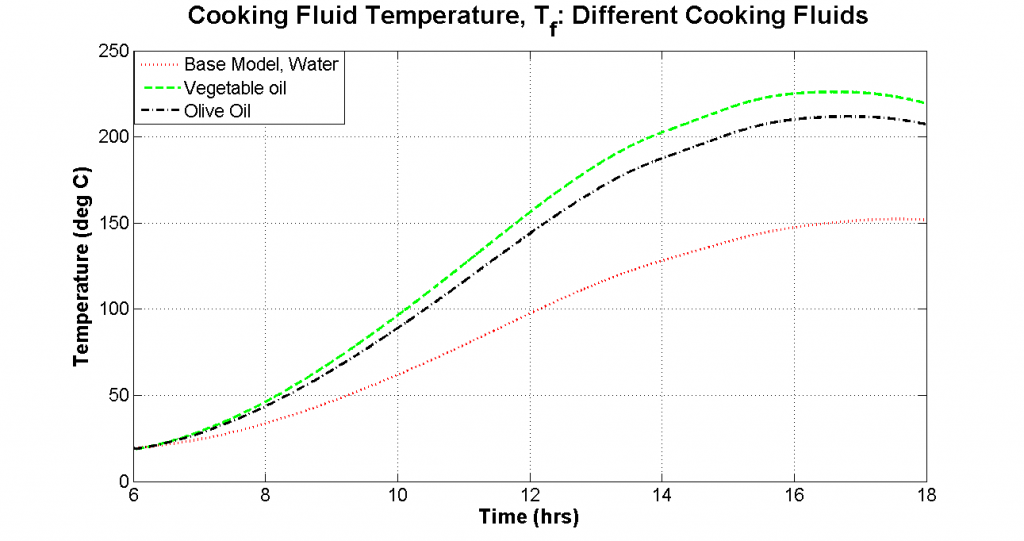## Conclusion

By comparing cooking fluid temperature, it was found that applying solar absorptive coatings on the cooking vessel has significant impact on the performance of the solar cooker. Although black paint can increase the absorptivity of the cooking vessel, multi-layer commercial absorptive coatings ensure maximum absorptivity with minimal reflection.

External reflectors of the solar cooker do not need to be highly advanced as changing optical properties of a reflector has a small effect on performance. Designers should not invest too much effort in expensive reflectors. However, the tilt angle of the reflector should be kept at an angle greater than the sun altitude at solar noon to avoid self-shading. The operator should be provided resources to identify optimal tilt angles.

In addition to solar absorptive coatings, it was found that higher temperatures can be obtained by reducing the size and heat capacity of the cooking fluid, using pressure cookers, using solar PV glass and pre-heating the cooking fluid.

In future work, a physical prototype will be fabricated to validate the mathematical model. The research will be expanded into investigating the use of phase change materials as thermal storage media.